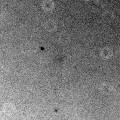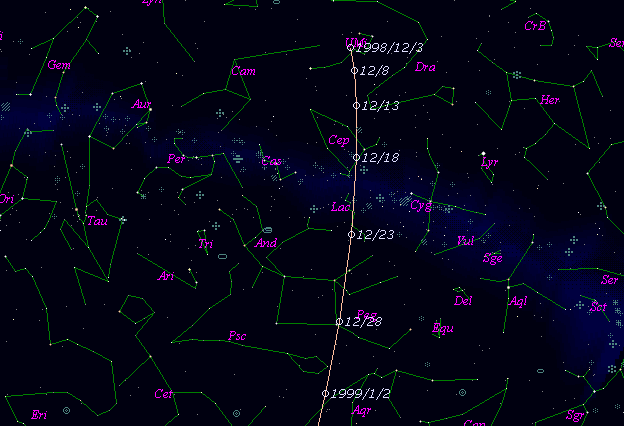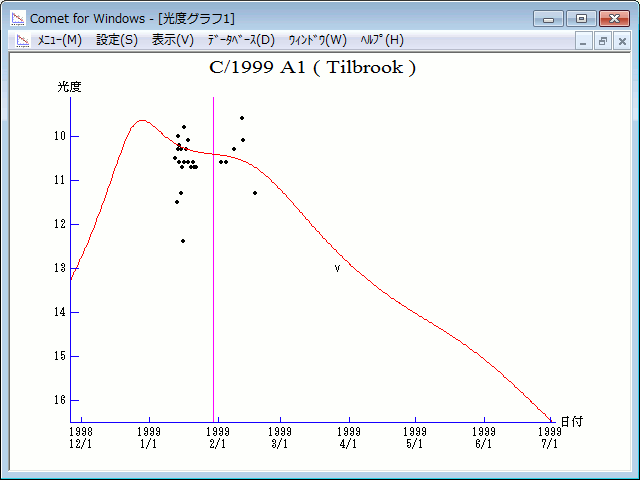# \$B%F%#%k%V%k%C%/WB@1(B

C/1999 A1 ( Tilbrook )

 English version Home page Updated on July 11, 1999###\$B%W%m%U%#!<%k(B

 \$BH/8+F|(B 1999\$BG/(B 1\$B7n(B12\$BF|(B \$BH/8+8wEY(B 10.5\$BEy(B \$BH/8+ Justin Tilbrook (Clare, South Australia)

###\$B###\$B50F;MWAG(B

```   The following improved orbital elements by Kenji Muraoka, are
from 64 observations  1999 Jan. 13  to  Feb. 25, perturbations
by 9 Planets, Moon and 5 minor planets were taken into account.
The mean residual is +/- 0.67 arc seconds.

Epoch  =  1999 Jan  = 2451200.5
T  =  1999 Jan. 29.65791       +/- 0.00058 (m.e.) TT
Peri. =  232.67071                +/- 0.00076
Node  =  259.12246                +/- 0.00062   (2000.0)
Incl. =   89.48108                +/- 0.00091
q  =    0.7307403              +/- 0.0000029 AU
e  =    0.9958512              +/- 0.0000423
1/a  =   +0.0056775              +/- 0.0000579 1/AU
( P  =   2338 years )
```

###\$B@1?^(B###\$B8wEYJQ2=(B

```        m1 = 12.3 + 5 log\$B&\$(B + 10.0 log r  [ ,0]  (              \$B!A(B1999\$BG/(B 1\$B7n(B29\$BF|(B)
m1 = 11.2 + 5 log\$B&\$(B + 10.0 log r  [0, ]  (1999\$BG/(B 1\$B7n(B29\$BF|!A(B              )
```##### \$B50F;MWAG\$OB<2,7r<#;a\$N7W;;\$K\$h\$k\$b\$N\$G\$9!#(B \$B@1?^\$O(B StellaNavigator Ver.2.0 for Windows (\$B%"%9%H%m%"!<%D(B \$BJTCx(B / \$B%"%9%-!<=PHG6I4)(B) \$B\$G:n@.\$7\$?\$b\$N\$G\$9!#(B \$B8wEY%0%i%U\$O(BComet for Windows\$B\$G:n@.\$7\$?\$b\$N\$G\$9!#(B